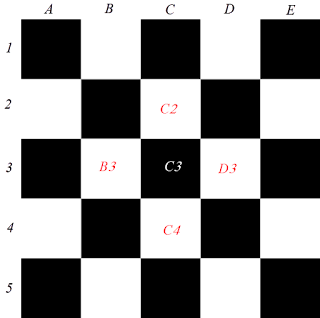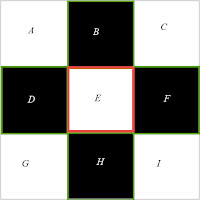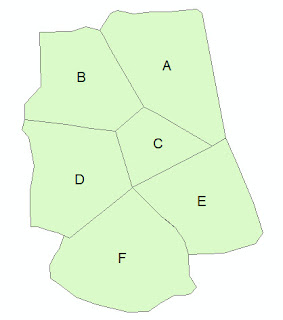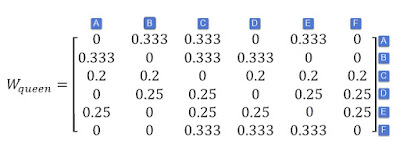## Introduction

This lesson will guide you on how to generate Spatial Weight Matrix using ArcGIS and PySAL.

## What is the spatial weights matrix (SWM)?

It is a matrix of weights (W) expressing the spatial relationships among the spatial units (features). In its simplest forms, spatial weight between feature i and feature j takes value of 1 (i.e. wij=1) when they are neighbours (e.g. have common borders); and it will take a value of zero (wij=0) otherwise. This way in estimating the weights is referred to contiguity-based weighting. The process of weighting is repeated for each pair of features. So, If we have n features, then the spatial weights matrix would be of size nxn
Figure(1) shows the structure of the spatial weight matrix. We will see some examples at the end of this post.Figure (1): Structure of Spatial Weight Matrix (SWM) (source: Luc Anselin Lectures, 2016)

SWM plays an important role in modelling the spatial autocorrelation between the spatial units (features), and in applying spatial regression analysis. The weights reflect the spatial interaction among features. Neighbour features are more likely to affect and interact with each other than those which are far away from each other. This fact is expressed in different words by Geographer Waldo R. Tobler, who stated that:
Everything is related to everything else, but near things are more related than distant things.
So, SWM mainly describes how near or far each feature from others. This can be modelled using distance or contiguity measures.Figure (2): Spatial Units (features) distributed as chess board
In Figure (2), If the feature C3 has high values of CO2 concentration, It is more likely that features C2, D3, C4, and B3 have similar (i.e. high values) as well. This is because the four features have common edges with C3, and thus spatial interaction would be highly expected.  However, that is not always the case. In practice, we sometimes find the neighbour features have dissimilar values. In other words, If a feature has high value, the neighbours may have low values. To measure and quantify such similarities and dissimilarities among features through the space, we need to estimate the correlation of the variable (e.g. CO2 Concentration) with itself through space. This is referred to spatial autocorrelation. If similar values occur near each other, then we have positive spatial autocorrelation. On the other hand, if dissimilar values occur near each other, then we have negative spatial autocorrelation. We will cover the topic of spatial autocorrelation in a separate post; and we will learn different indicators (e.g. Moran's I) to estimate the spatial autocorrelation.
For now, we should know that for estimating spatial autocorrelation, we need to conceptualise the spatial relationships among features. This can be accomplished by constructing the spatial weights matrix.

There are many methods for generating the spatial weights matrix. You can refer to this web page for more information on those methods. In this post, we will focus on contiguity-based spatial weights matrix.

### What is Contiguity?

Contiguity among features means the features have common borders. We have three types of contiguity:
1. Rook Contiguity: the features share common edges
2. Bishop Contiguity: the features share common vertices (corners)
3. Queen Contiguity: the feature share common edges and corners.
Figure (3) shows the difference between the three types.Figure (3): Three types of contiguity (source: Luc Anselin Lectures, 2016)

For example, If we have 9 spatial units (features) taking the form of chess board as shown in Figure(4), then the spatial weight matrix would be like the one shown in Figure(5).Figure (4): The feature E has four neighbours based on Rook ContiguityFigure(5): The spatial weight matrix for the features of Figure(4)

Let's do some examples. We will generate the spatial matrix for the features in Figure (6) (artificial example) manually and using ArcGIS and PySAL.Figure (6): The six features for which SWM to be constructed
The spatial weights matrix can be constructed manually as shown below:Figure (7): Spatial Weight Matrix based on Queen Contiguity (left) and Rook Contiguity (right)
Before moving to the next section, There are two notes about the previous spatial weights matrices:
1. We need sometimes to standardise SWM by applying row standardisation. Each neighbour weight for a feature is divided by the sum (row sum) of weights of all neighbours of that feature. For example, by looking again into Wrook in Figure(7), we find that feature C has four neighbours and the weight for each is equal to one: w=1; so the standardised weight will be equal 1/4=0.25. Figure(8) shows the standardised weights matrix based on queen contiguity for our example. Row standardisation is required for different reasons. You can refer  to this web page for more information.
2. We can derive some descriptive statistics from SWM, in order to get more insight into the spatial structure of the features. The following stats are derived from the queen-based SWM in figure(7):
• Number of features = 6
• Percentage of spatial connectivity = (Number of non-zero weights) / (Number of all weights) x 100 = 22/36 x 100 = 61.11%
• Average Number of neighbours = Sum of  (# neighbours for each feature)  for all features / number of features = (3+3+5+4+3+3)/6 = 22/6 = 3.67 neighbours.
• Minimum number of neighbours = 3
• Maximum number of neighbours = 5Figure (8): Standardised Queen Contiguity SWM

## Generating the spatial weight matrix in ArcGIS and PySAL

### Contiguity-based Spatial Weight Matrix using ArcGIS:

ArcGIS provides a geoprocessing tool which can generate spatial weight matrix using Contiguity methods and Distance methods as well. This tool is Generate Spatial Weights Matrix . This tool takes the features as shapefile or feature class, and outputs the spatial weights as a binary file with *.swm extension.
To view the features, neighbours, and their weights, we need to convert the binary *.swm file a table using the tool: Convert Spatial Weights Matrix to Table.

Below is a short video demonstrating how ArcGIS is used to generate the contiguity-based spatial matrix.

### Contiguity-based Spatial Weight Matrix using PySAL

PySAL is an open source library of spatial analysis functions written in Python intended to support the development of high level applications. PySAL is open source under the BSD License.

PySAL supports creation of the spatial weights matrices through three different general types:
• Contiguity-based weights
• Distance-based weights
• kernel weights
We are concerned with Contiguity-based weights. The three types are subclasses of the general and main class for spatial weights : pysal.weights.W. The class W has many attributes which uncover the properties of the spatial weights matrix, and let you manipulate and analyse the weights for deeper spatial analysis.

Moreover, PySAL provides you with several functions to create spatial weights matrices directly from the shapefile.
Please refer to the main website of PySAL for more details, documentation, and tutorials.

Here is Python script which utilises PySAL to generate contiguity-based (Queen and Rook) Spatial Weights Matrices.

 ``` 1 2 3 4 5 6 7 8 9 10 11 12 13 14 15 16 17 18 19 20 21 22 23 24 25 26 27 28 29 30 31``` ```# -*- coding: utf-8 -*- """ Created on Sat Jan 26 16:51:39 2019 @author: Mohammed Alhessi """ # import the required modules import pysal # create the spatial weights matrix from shapefile # There are many ways to do that. Below is the simplest one # path to shapefile shp = r"C:\Users\0GEOMATICS\AI\PROJECTS\XYZ GEOLOG\Spatial Weights\GIS\SHP\Spatial_Units.shp" # create Queen Contiguity-based Spatial Weight Matrix (SWM) swm_queen = pysal.queen_from_shapefile(shp) # create Queen Contiguity-based Spatial Weight Matrix swm_rook = pysal.rook_from_shapefile(shp) # output results for Queen SWM print("The Queen Contiguity Spatial Weight Matrix is:\n") print(swm_queen.full()) print("Number of features: " + str(swm_queen.n)) print("Percentage of Spatial Connectivity: " + str(swm_queen.pct_nonzero)) print("Average Number of Neighbors: " + str(swm_queen.mean_neighbors)) print("Minimum Number of Neighbors: " + str(swm_queen.min_neighbors)) print("Maximum Number of Neighbors: " + str(swm_queen.max_neighbors)) ```

After running the above code, the results would be as shown in the following figure:Figure (9): Output of the python script